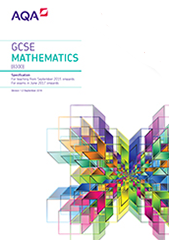# Specification at a glance

## Assessments

For 2023, a formulae sheet will be provided for exams. This will be added as an insert with every question paper, or you can download the formulae sheet now.

GCSE Mathematics has a Foundation tier (grades 1 – 5) and a Higher tier (grades 4 – 9). Students must take three question papers at the same tier. All question papers must be taken in the same series.

The information in the table below is the same for both Foundation and Higher tiers.

The Subject content section shows the content that is assessed in each tier.

Paper 1: non-calculator

What's assessed

Content from any part of the specification may be assessed

How it's assessed

• written exam: 1 hour 30 minutes
• 80 marks
• non-calculator
• 33⅓% of the GCSE Mathematics assessment

Questions

A mix of question styles, from short, single-mark questions to multi-step problems. The mathematical demand increases as a student progresses through the paper.Paper 2: calculator

What's assessed

Content from any part of the specification may be assessed

How it's assessed

• written exam: 1 hour 30 minutes
• 80 marks
• calculator allowed
• 33⅓% of the GCSE Mathematics assessment

Questions

A mix of question styles, from short, single-mark questions to multi-step problems. The mathematical demand increases as a student progresses through the paper.Paper 3: calculator

What's assessed

Content from any part of the specification may be assessed

How it's assessed

• written exam: 1 hour 30 minutes
• 80 marks
• calculator allowed
• 33⅓% of the GCSE Mathematics assessment

Questions

A mix of question styles, from short, single-mark questions to multi-step problems. The mathematical demand increases as a student progresses through the paper.